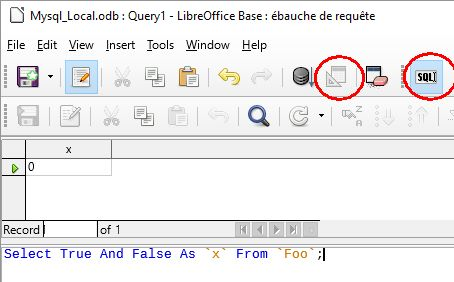# MariaDB & MySQL SQL syntax: using boolean AND or OR in Base queries

In Base query design what’s the SQL syntax for `AND` and `OR` when connected to MySQL?

Typical usage examples:

``````Select True And False As `x` From `Foo`;
Select 1=1 And 2=2 As `x` From `Foo`;
Select if(1=1 And 2=2, 5, 6) As `x` From `Foo`;
Select if((1=1) And (2=2), 5, 6) As `x` From `Foo`;
``````

I’ve tried everything I can think of including `...AND(1=1; 2=2)...`, but keep getting a syntax error.

? Also, why is it again that there is this SQL interpretation process that goes on?

BTW, in the Tools | SQL window (uninterpreted) the following work as expected:

``````SELECT 1=1 And 2=2 As `x` From `Foo`;
SELECT (1=1 And 2=2) As `x` From `Foo`;
SELECT (1=1) And (2=2) As `x` From `Foo`;
SELECT if((1=1) And (2=2),5,6) As `x` From `Foo`;
``````

Also of note is that

``````SELECT 1=1 As `x` ...
``````

does not work as expected. Instead you must write

``````SELECT if(1=1,true, false) As `x` ..
``````

Thanks.

Using LO 5.3.3.2

If starting from the design view, click the toolbar icon to Switch Design View On/Off. Then click the toolbar icon to Run SQL command directly to avoid the interpretation process. Now everything works as expected.Note: I do not know what benefit the interpretation process provides. Invariably, my queries are run directly. This makes it simple because the same behavior occurs from a MySQL prompt as from a Base query.

The interpreter preforms some basic syntax verification before passing on. However, this is for generally for HSQL v1.8. It does provide for a user input parameter such as `:enter_something`. With Run directly on, this is not available.

Thanks guys! Also just created this enhancement suggestion: https://bugs.documentfoundation.org/show_bug.cgi?id=112801

Here are some workaround’s:

OR:

`||` provides OR functionality, i.e. it returns true for

True || True
True || False
False || True, but false for
False || False.

NOT:

`If(condition,FALSE,TRUE)` – This is messy, but it works, i.e. if condition is true this returns false.

AND:

This boolean identity can then be used to produce AND from OR:

x AND y = NOT (NOT x OR Not y)

Therefore

``````If( If(x,FALSE,TRUE) || If(y,FALSE,TRUE), FALSE, TRUE)
``````

For example:

``````If( If( x=1, FALSE, TRUE ) || If( y=1, FALSE, TRUE ), FALSE, TRUE )
``````

And this works in MySQL, but oh, what a mess.

As I recall, in HSQLDB this requires CASEWHEN rather than simply IF. But unfortunately, `||` does not provide an OR function in HSQLDB.

From the HSQLDB1.8 User Guide:

SQL Expression

[NOT] condition [{ OR | AND }
condition]"

But I can’t seem to get it to work, not even in HSQLDB.

If testing for nulls:

Try `concat([list of your values])`, and then test for `IS NOT EMPTY` - often much simpler than using `if(isnull(...), ..)`